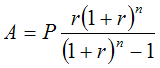Glossary definition

# What is amortization?

## Amortization

In accounting, amortization refers to the practice of spreading out the expense of an asset over a period of time that typically coincides with the principle asset’s useful life. Amortizing an expense is useful in determining the true benefit of a large expense as it generates revenue over time. The amounts of each increment of a spread-out expense as reported on a company’s financials define amortization expenses. Amortization practices reflect a more accurate cost of doing business in a company’s financial reporting, as the benefits of an initial expense may continue long after the initial report of that expense.

A broader amortization definition includes the process of gradually paying off the loan balance over a set amount of monthly payments and in fixed increments, commonly seen in home mortgages and auto loans.

## What is the difference between depreciation and amortization?

The difference between amortization and depreciation is that amortization is used for intangible assets, while depreciation is used for tangible assets.

## What formula do I use to calculate amortization?

Here is the formula to calculate amortization.• A = payment Amount per period
• P = initial Principal (loan amount)
• r = fixed interest rate per period
• n = total number of payments or periods